Скачать презентацию 의료기기를 위한 공학이론 Linear Systems Control Professor

75e6d17896ab6932e991589a290675d8.ppt

• Количество слайдов: 60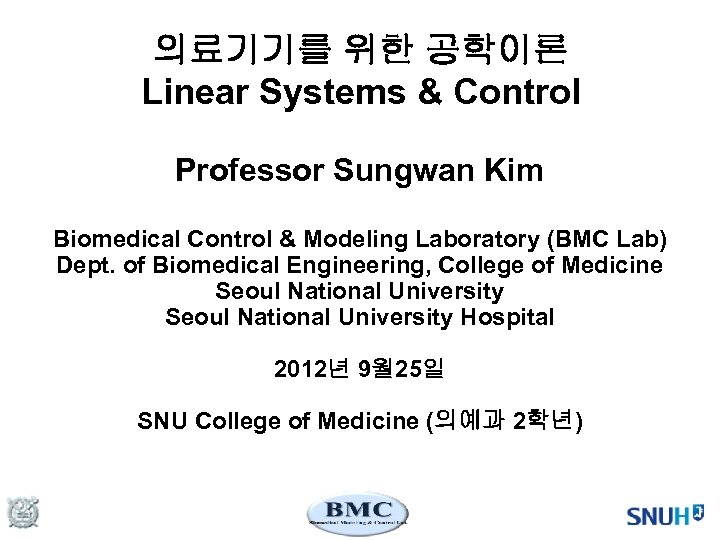의료기기를 위한 공학이론 Linear Systems & Control Professor Sungwan Kim Biomedical Control & Modeling Laboratory (BMC Lab) Dept. of Biomedical Engineering, College of Medicine Seoul National University Hospital 2012년 9월 25일 SNU College of Medicine (의예과 2학년)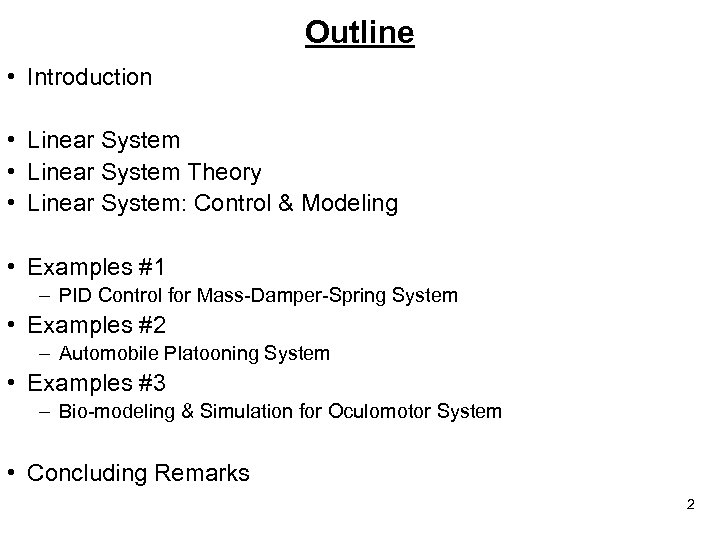Outline • Introduction • Linear System Theory • Linear System: Control & Modeling • Examples #1 – PID Control for Mass-Damper-Spring System • Examples #2 – Automobile Platooning System • Examples #3 – Bio-modeling & Simulation for Oculomotor System • Concluding Remarks 2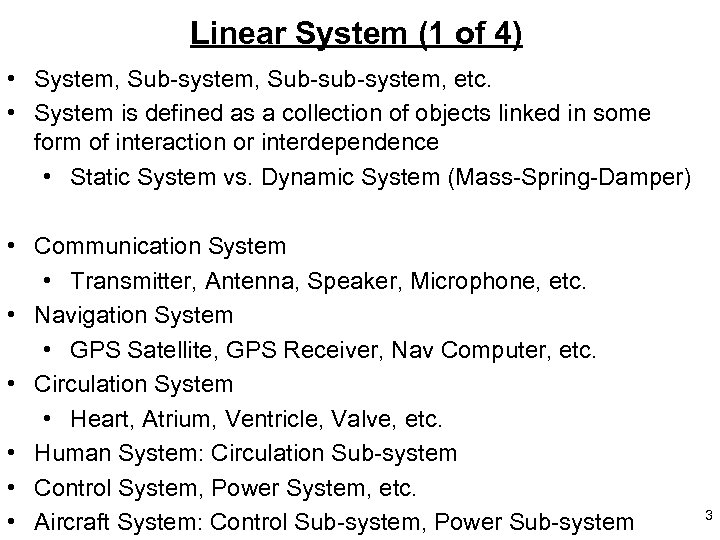Linear System (1 of 4) • System, Sub-sub-system, etc. • System is defined as a collection of objects linked in some form of interaction or interdependence • Static System vs. Dynamic System (Mass-Spring-Damper) • Communication System • Transmitter, Antenna, Speaker, Microphone, etc. • Navigation System • GPS Satellite, GPS Receiver, Nav Computer, etc. • Circulation System • Heart, Atrium, Ventricle, Valve, etc. • Human System: Circulation Sub-system • Control System, Power System, etc. • Aircraft System: Control Sub-system, Power Sub-system 3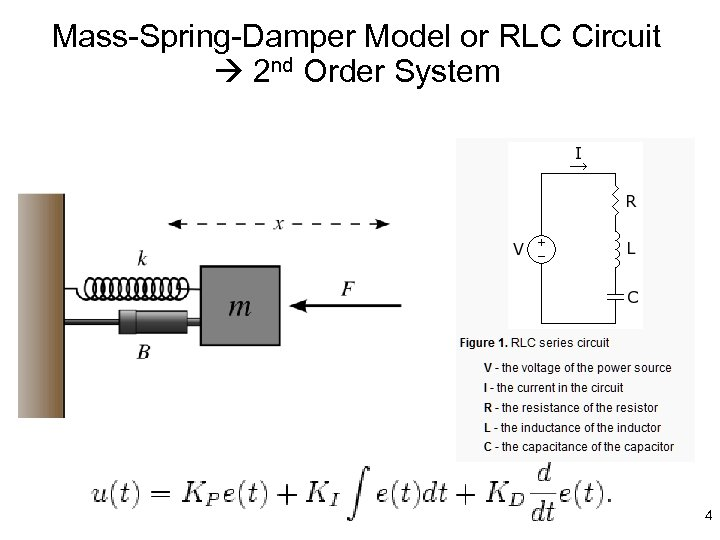Mass-Spring-Damper Model or RLC Circuit 2 nd Order System 4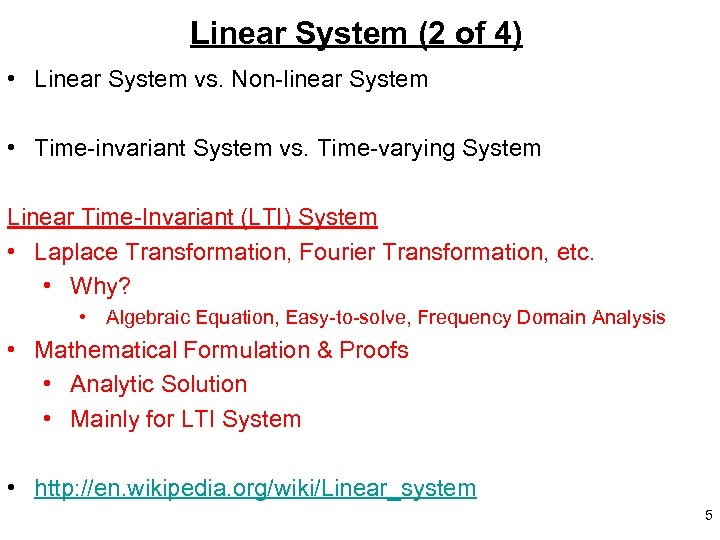Linear System (2 of 4) • Linear System vs. Non-linear System • Time-invariant System vs. Time-varying System Linear Time-Invariant (LTI) System • Laplace Transformation, Fourier Transformation, etc. • Why? • Algebraic Equation, Easy-to-solve, Frequency Domain Analysis • Mathematical Formulation & Proofs • Analytic Solution • Mainly for LTI System • http: //en. wikipedia. org/wiki/Linear_system 5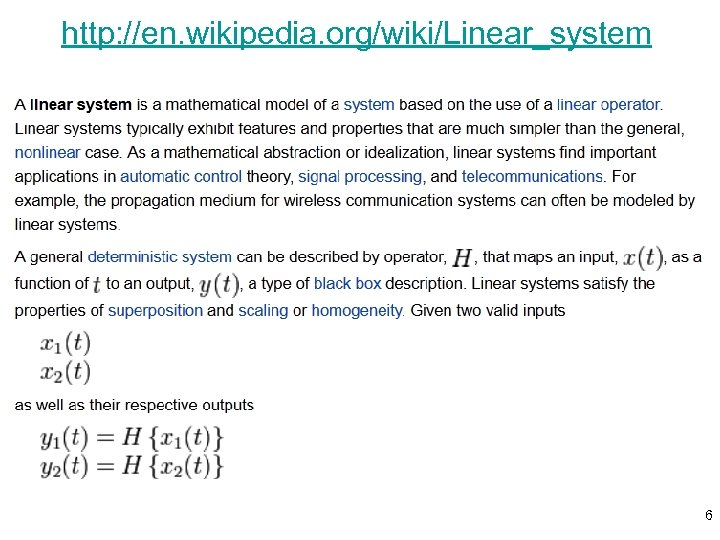http: //en. wikipedia. org/wiki/Linear_system 6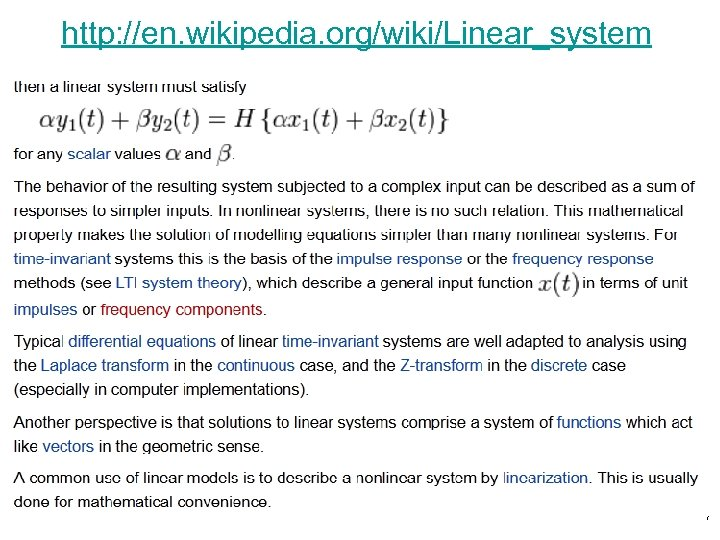http: //en. wikipedia. org/wiki/Linear_system 7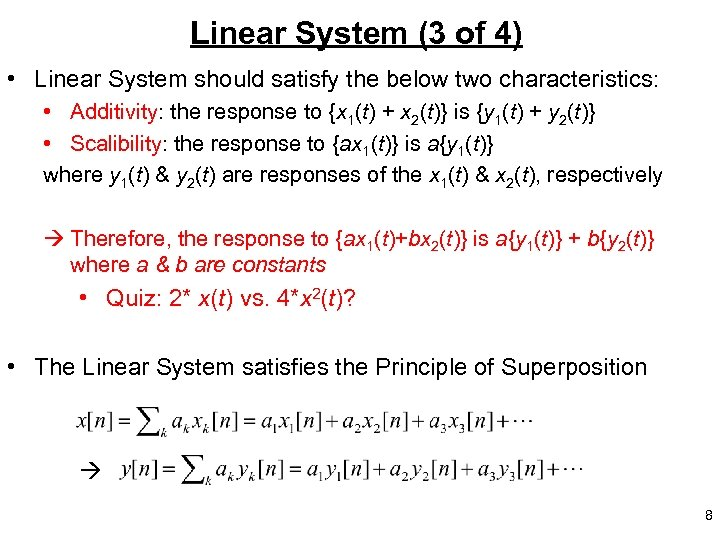Linear System (3 of 4) • Linear System should satisfy the below two characteristics: • Additivity: the response to {x 1(t) + x 2(t)} is {y 1(t) + y 2(t)} • Scalibility: the response to {ax 1(t)} is a{y 1(t)} where y 1(t) & y 2(t) are responses of the x 1(t) & x 2(t), respectively Therefore, the response to {ax 1(t)+bx 2(t)} is a{y 1(t)} + b{y 2(t)} where a & b are constants • Quiz: 2* x(t) vs. 4*x 2(t)? • The Linear System satisfies the Principle of Superposition 8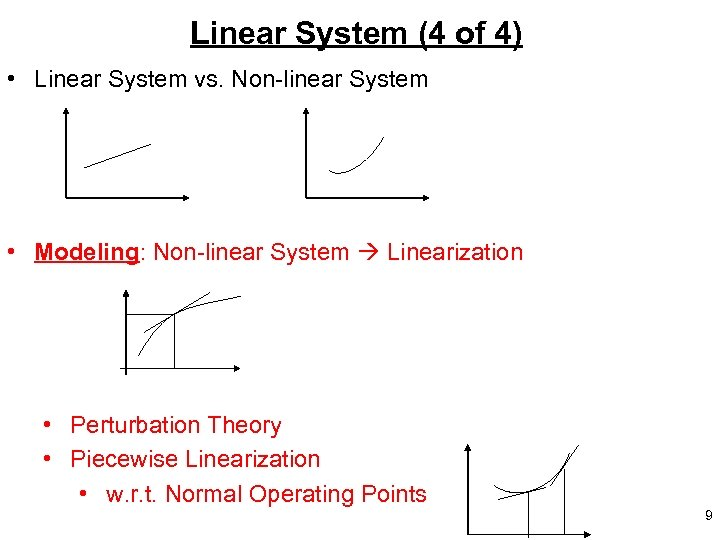Linear System (4 of 4) • Linear System vs. Non-linear System • Modeling: Non-linear System Linearization • Perturbation Theory • Piecewise Linearization • w. r. t. Normal Operating Points 9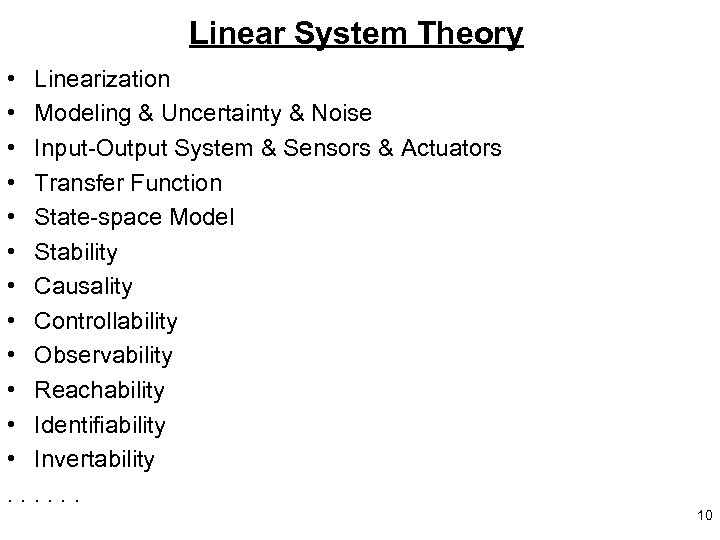Linear System Theory • Linearization • Modeling & Uncertainty & Noise • Input-Output System & Sensors & Actuators • Transfer Function • State-space Model • Stability • Causality • Controllability • Observability • Reachability • Identifiability • Invertability. . . 10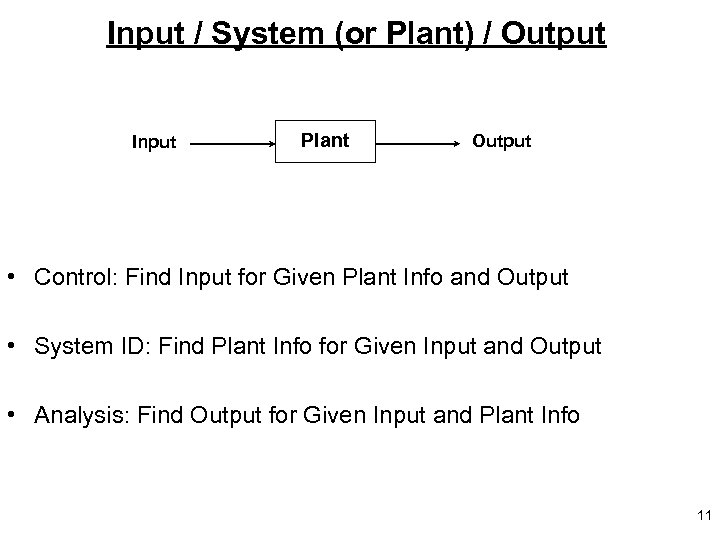Input / System (or Plant) / Output Input Plant Output • Control: Find Input for Given Plant Info and Output • System ID: Find Plant Info for Given Input and Output • Analysis: Find Output for Given Input and Plant Info 11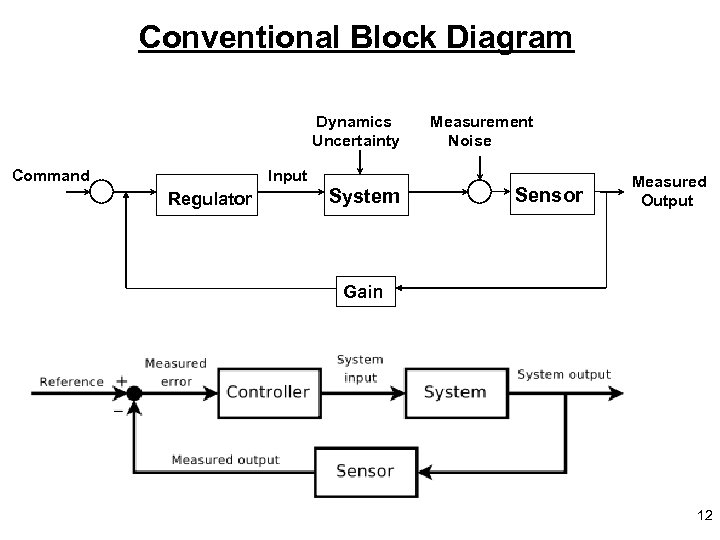Conventional Block Diagram Dynamics Uncertainty Command Input Regulator System Measurement Noise Sensor Measured Output Gain 12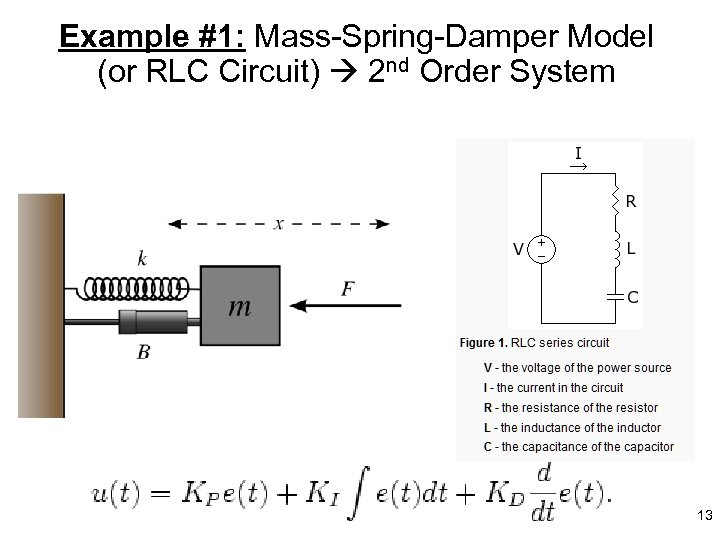Example #1: Mass-Spring-Damper Model (or RLC Circuit) 2 nd Order System 13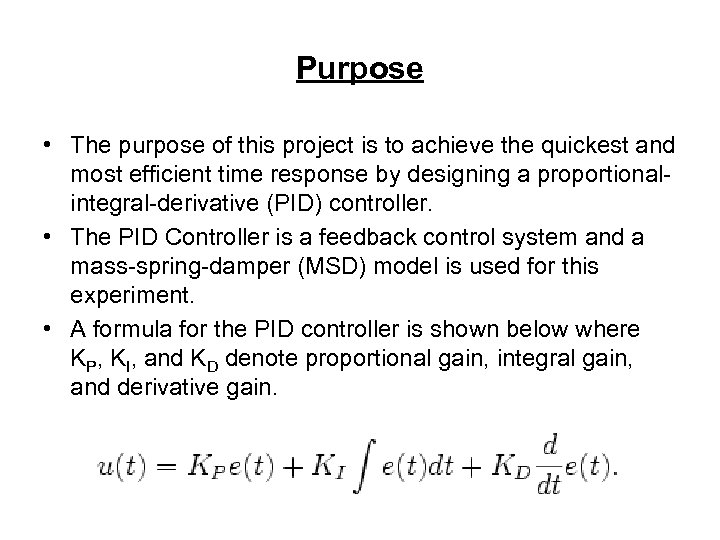Purpose • The purpose of this project is to achieve the quickest and most efficient time response by designing a proportionalintegral-derivative (PID) controller. • The PID Controller is a feedback control system and a mass-spring-damper (MSD) model is used for this experiment. • A formula for the PID controller is shown below where KP, KI, and KD denote proportional gain, integral gain, and derivative gain.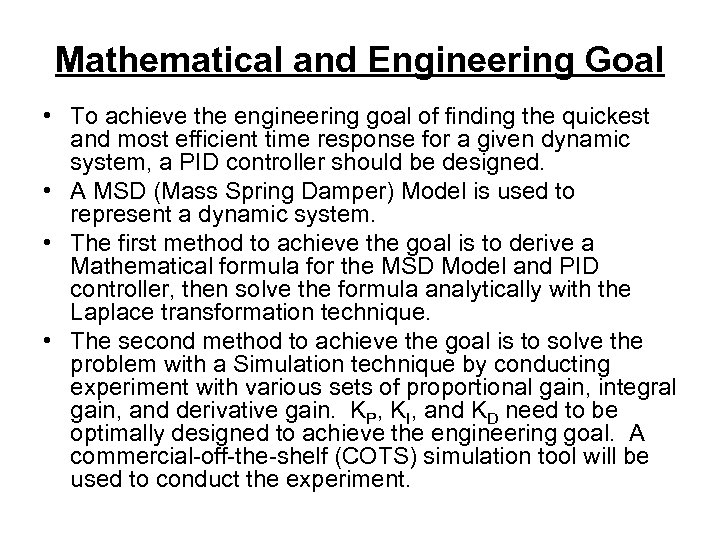Mathematical and Engineering Goal • To achieve the engineering goal of finding the quickest and most efficient time response for a given dynamic system, a PID controller should be designed. • A MSD (Mass Spring Damper) Model is used to represent a dynamic system. • The first method to achieve the goal is to derive a Mathematical formula for the MSD Model and PID controller, then solve the formula analytically with the Laplace transformation technique. • The second method to achieve the goal is to solve the problem with a Simulation technique by conducting experiment with various sets of proportional gain, integral gain, and derivative gain. KP, KI, and KD need to be optimally designed to achieve the engineering goal. A commercial-off-the-shelf (COTS) simulation tool will be used to conduct the experiment.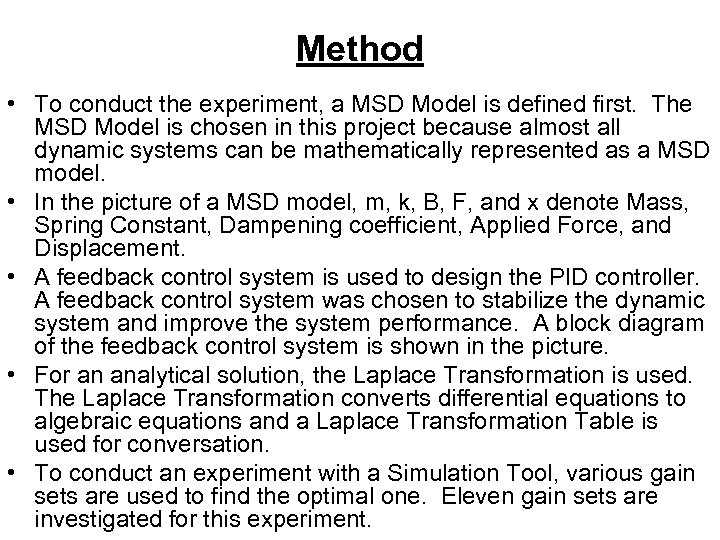Method • To conduct the experiment, a MSD Model is defined first. The MSD Model is chosen in this project because almost all dynamic systems can be mathematically represented as a MSD model. • In the picture of a MSD model, m, k, B, F, and x denote Mass, Spring Constant, Dampening coefficient, Applied Force, and Displacement. • A feedback control system is used to design the PID controller. A feedback control system was chosen to stabilize the dynamic system and improve the system performance. A block diagram of the feedback control system is shown in the picture. • For an analytical solution, the Laplace Transformation is used. The Laplace Transformation converts differential equations to algebraic equations and a Laplace Transformation Table is used for conversation. • To conduct an experiment with a Simulation Tool, various gain sets are used to find the optimal one. Eleven gain sets are investigated for this experiment.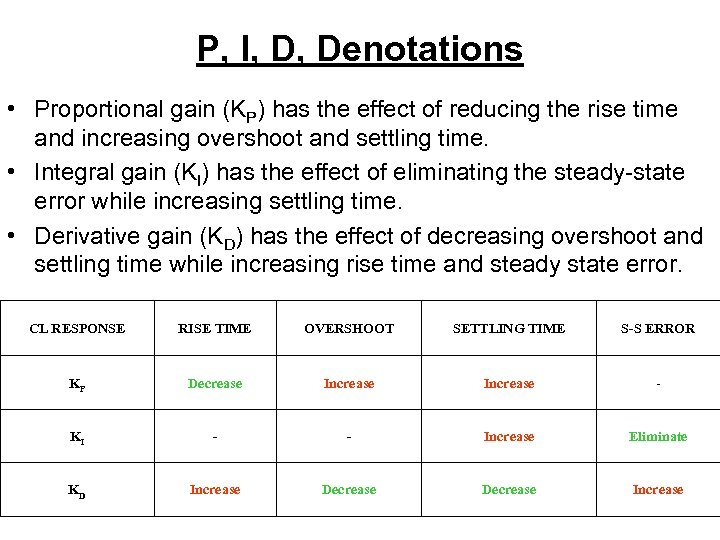P, I, D, Denotations • Proportional gain (KP) has the effect of reducing the rise time and increasing overshoot and settling time. • Integral gain (KI) has the effect of eliminating the steady-state error while increasing settling time. • Derivative gain (KD) has the effect of decreasing overshoot and settling time while increasing rise time and steady state error. CL RESPONSE RISE TIME OVERSHOOT SETTLING TIME S-S ERROR KP Decrease Increase - KI - - Increase Eliminate KD Increase Decrease Increase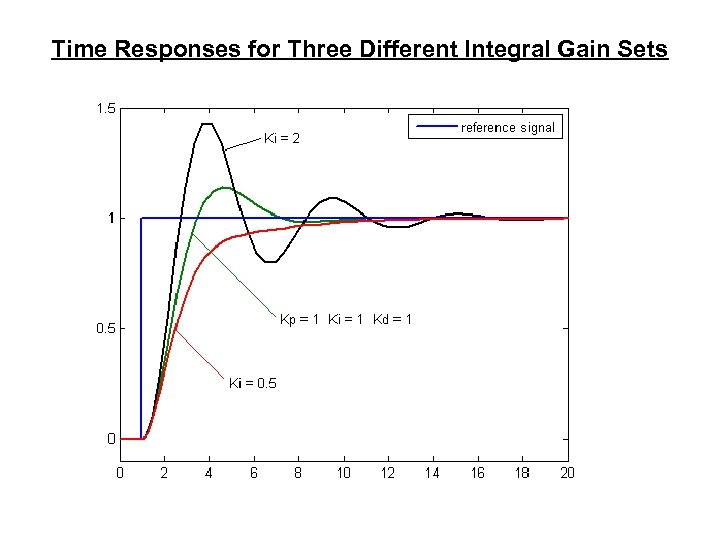Time Responses for Three Different Integral Gain Sets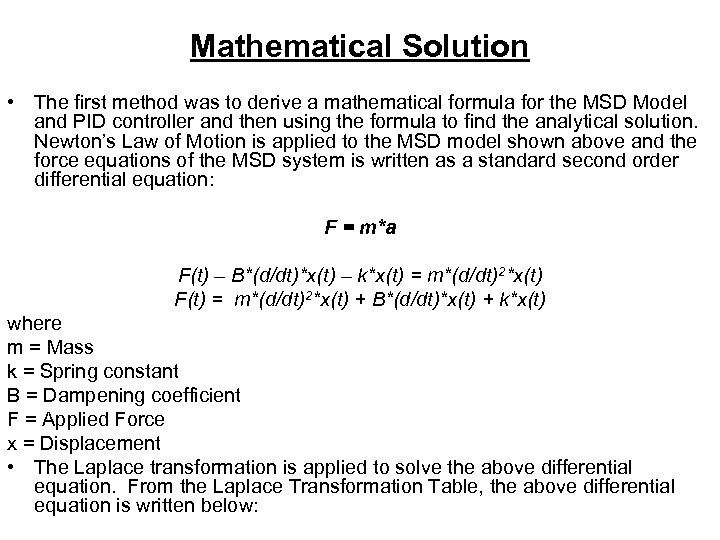Mathematical Solution • The first method was to derive a mathematical formula for the MSD Model and PID controller and then using the formula to find the analytical solution. Newton’s Law of Motion is applied to the MSD model shown above and the force equations of the MSD system is written as a standard second order differential equation: F = m*a F(t) – B*(d/dt)*x(t) – k*x(t) = m*(d/dt)2*x(t) F(t) = m*(d/dt)2*x(t) + B*(d/dt)*x(t) + k*x(t) where m = Mass k = Spring constant B = Dampening coefficient F = Applied Force x = Displacement • The Laplace transformation is applied to solve the above differential equation. From the Laplace Transformation Table, the above differential equation is written below: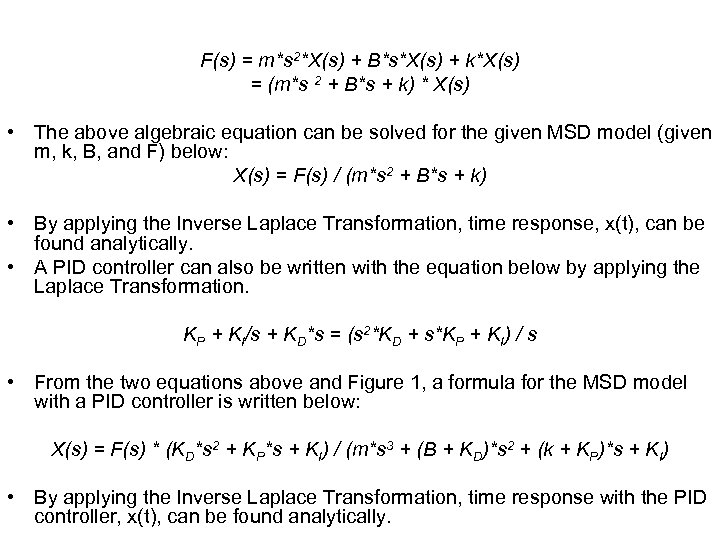F(s) = m*s 2*X(s) + B*s*X(s) + k*X(s) = (m*s 2 + B*s + k) * X(s) • The above algebraic equation can be solved for the given MSD model (given m, k, B, and F) below: X(s) = F(s) / (m*s 2 + B*s + k) • By applying the Inverse Laplace Transformation, time response, x(t), can be found analytically. • A PID controller can also be written with the equation below by applying the Laplace Transformation. KP + KI/s + KD*s = (s 2*KD + s*KP + KI) / s • From the two equations above and Figure 1, a formula for the MSD model with a PID controller is written below: X(s) = F(s) * (KD*s 2 + KP*s + KI) / (m*s 3 + (B + KD)*s 2 + (k + KP)*s + KI) • By applying the Inverse Laplace Transformation, time response with the PID controller, x(t), can be found analytically.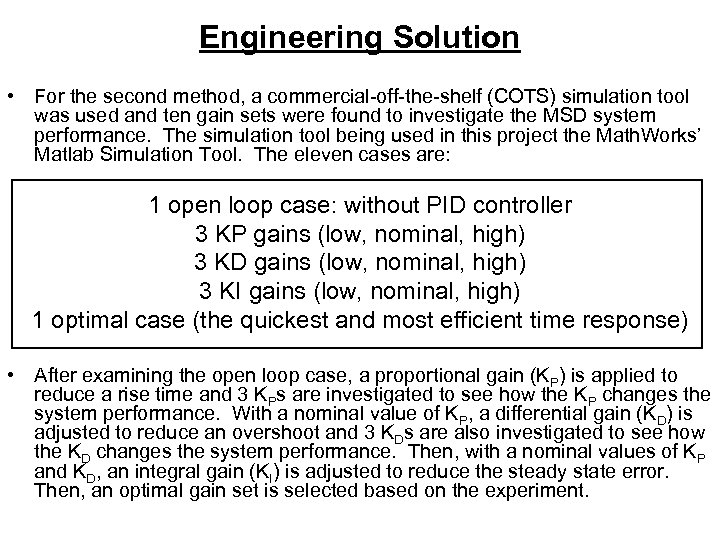Engineering Solution • For the second method, a commercial-off-the-shelf (COTS) simulation tool was used and ten gain sets were found to investigate the MSD system performance. The simulation tool being used in this project the Math. Works’ Matlab Simulation Tool. The eleven cases are: 1 open loop case: without PID controller 3 KP gains (low, nominal, high) 3 KD gains (low, nominal, high) 3 KI gains (low, nominal, high) 1 optimal case (the quickest and most efficient time response) • After examining the open loop case, a proportional gain (KP) is applied to reduce a rise time and 3 KPs are investigated to see how the KP changes the system performance. With a nominal value of KP, a differential gain (KD) is adjusted to reduce an overshoot and 3 KDs are also investigated to see how the KD changes the system performance. Then, with a nominal values of KP and KD, an integral gain (KI) is adjusted to reduce the steady state error. Then, an optimal gain set is selected based on the experiment.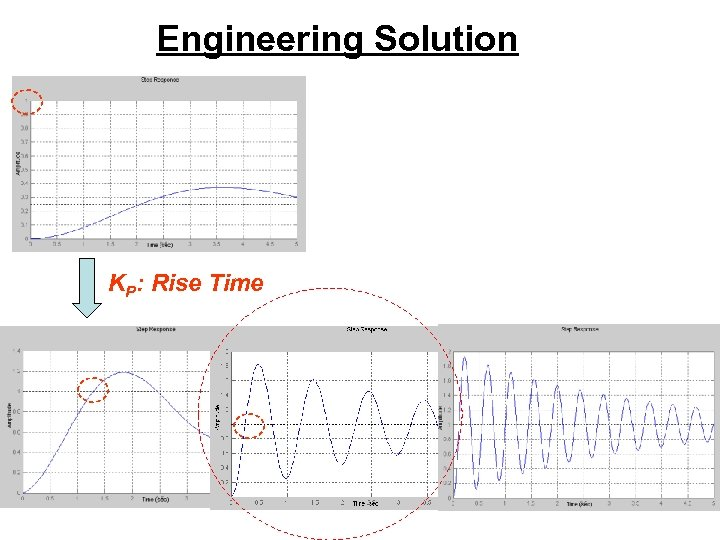Engineering Solution KP: Rise Time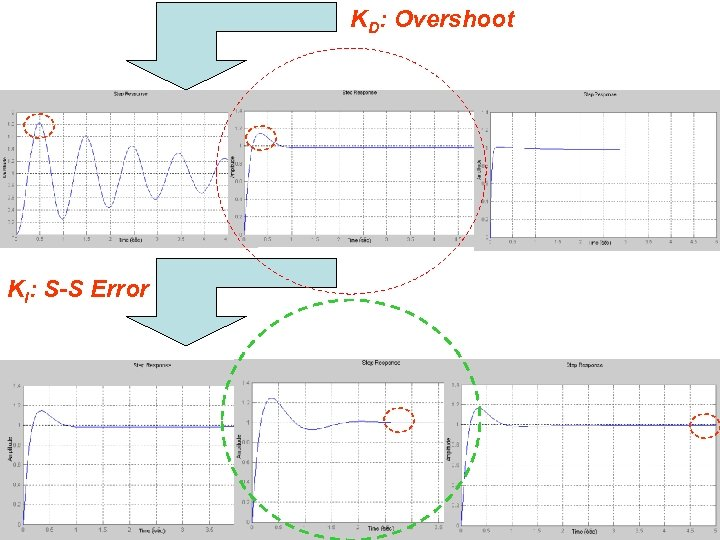KD: Overshoot KI: S-S Error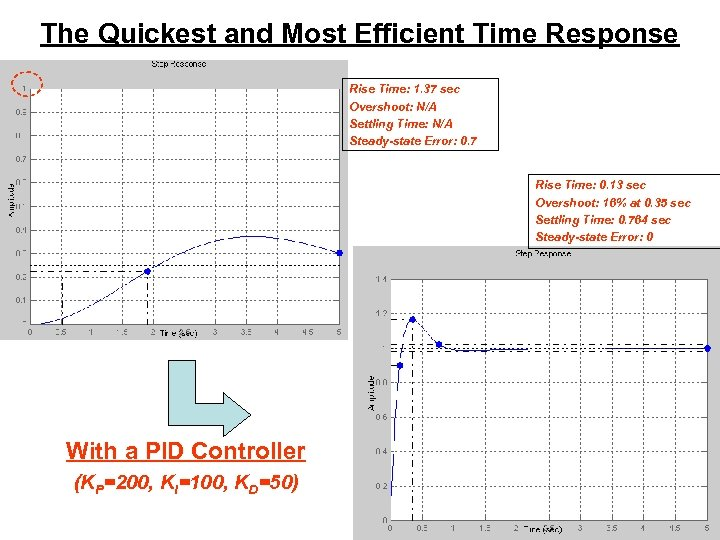The Quickest and Most Efficient Time Response Rise Time: 1. 37 sec Overshoot: N/A Settling Time: N/A Steady-state Error: 0. 7 Rise Time: 0. 13 sec Overshoot: 16% at 0. 35 sec Settling Time: 0. 764 sec Steady-state Error: 0 With a PID Controller (KP=200, KI=100, KD=50)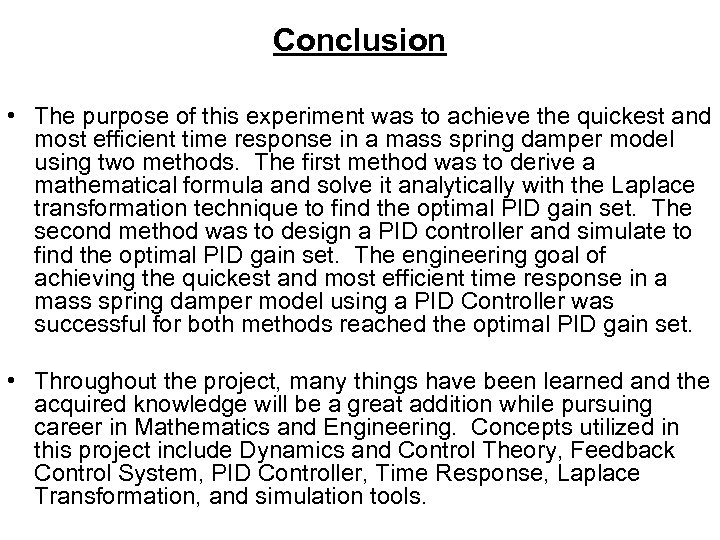Conclusion • The purpose of this experiment was to achieve the quickest and most efficient time response in a mass spring damper model using two methods. The first method was to derive a mathematical formula and solve it analytically with the Laplace transformation technique to find the optimal PID gain set. The second method was to design a PID controller and simulate to find the optimal PID gain set. The engineering goal of achieving the quickest and most efficient time response in a mass spring damper model using a PID Controller was successful for both methods reached the optimal PID gain set. • Throughout the project, many things have been learned and the acquired knowledge will be a great addition while pursuing career in Mathematics and Engineering. Concepts utilized in this project include Dynamics and Control Theory, Feedback Control System, PID Controller, Time Response, Laplace Transformation, and simulation tools.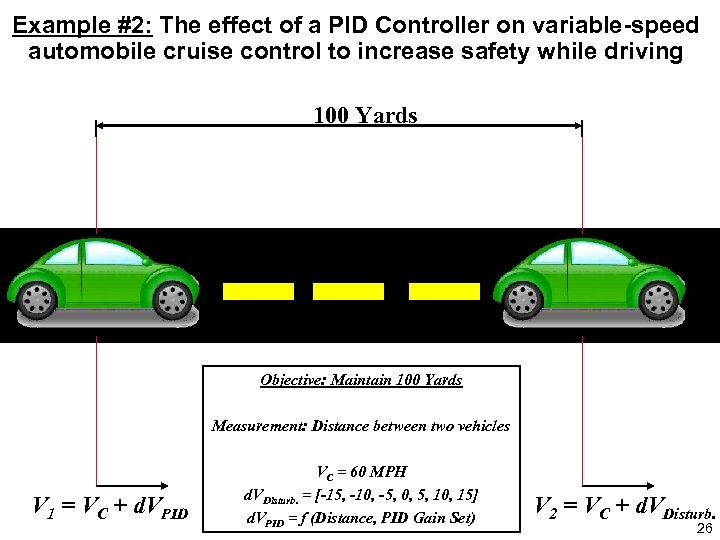Example #2: The effect of a PID Controller on variable-speed automobile cruise control to increase safety while driving 100 Yards Objective: Maintain 100 Yards Measurement: Distance between two vehicles V 1 = VC + d. VPID VC = 60 MPH d. VDisturb. = [-15, -10, -5, 0, 5, 10, 15] d. VPID = f (Distance, PID Gain Set) V 2 = VC + d. VDisturb. 26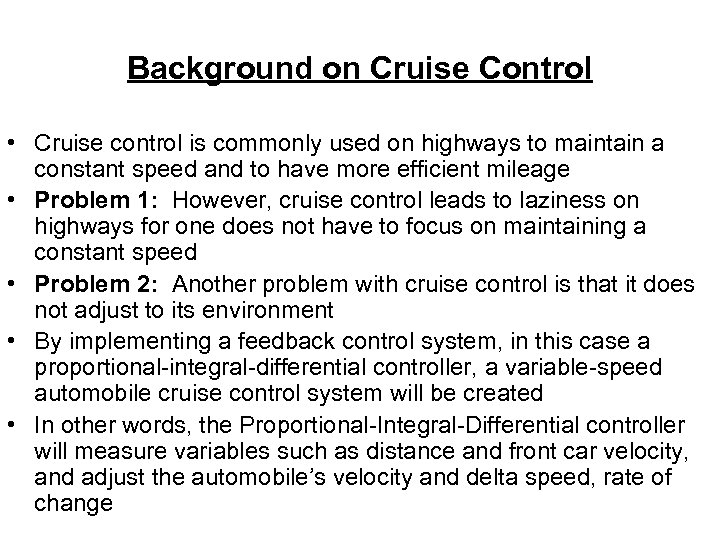Background on Cruise Control • Cruise control is commonly used on highways to maintain a constant speed and to have more efficient mileage • Problem 1: However, cruise control leads to laziness on highways for one does not have to focus on maintaining a constant speed • Problem 2: Another problem with cruise control is that it does not adjust to its environment • By implementing a feedback control system, in this case a proportional-integral-differential controller, a variable-speed automobile cruise control system will be created • In other words, the Proportional-Integral-Differential controller will measure variables such as distance and front car velocity, and adjust the automobile’s velocity and delta speed, rate of change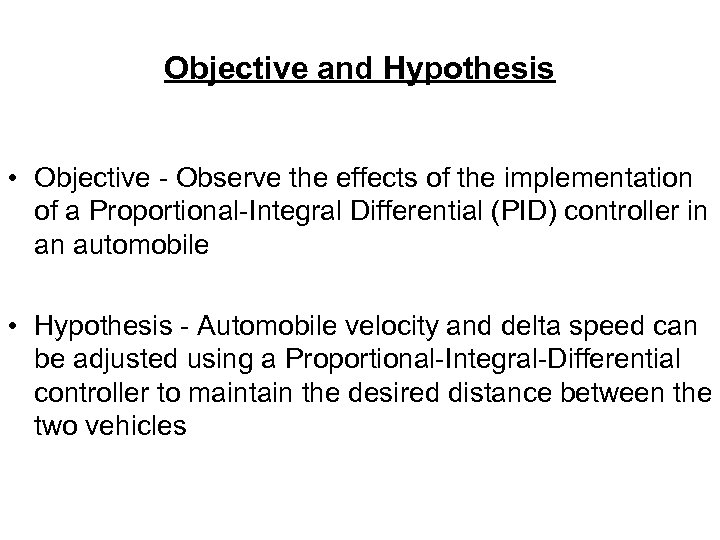Objective and Hypothesis • Objective - Observe the effects of the implementation of a Proportional-Integral Differential (PID) controller in an automobile • Hypothesis - Automobile velocity and delta speed can be adjusted using a Proportional-Integral-Differential controller to maintain the desired distance between the two vehicles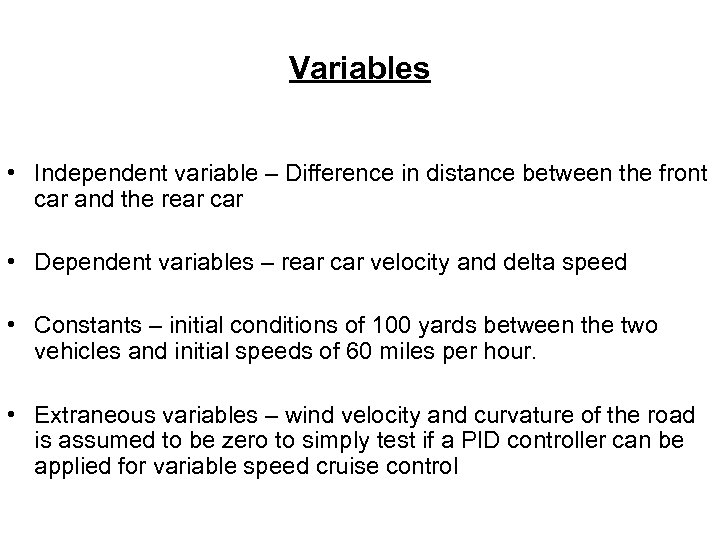Variables • Independent variable – Difference in distance between the front car and the rear car • Dependent variables – rear car velocity and delta speed • Constants – initial conditions of 100 yards between the two vehicles and initial speeds of 60 miles per hour. • Extraneous variables – wind velocity and curvature of the road is assumed to be zero to simply test if a PID controller can be applied for variable speed cruise control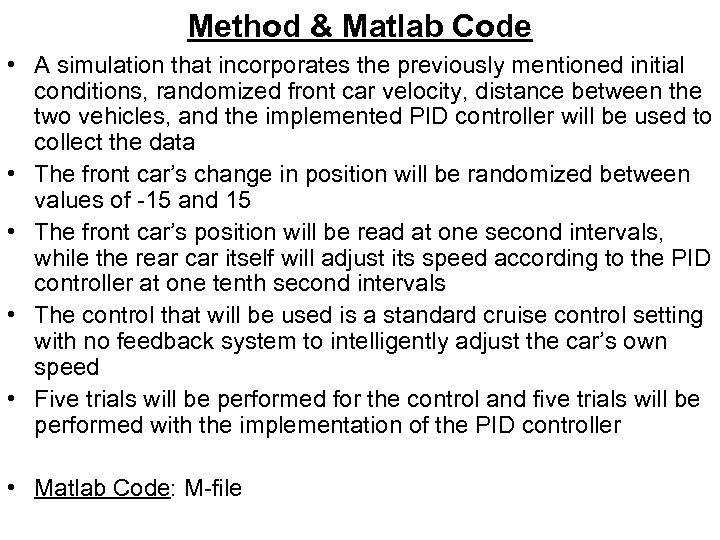Method & Matlab Code • A simulation that incorporates the previously mentioned initial conditions, randomized front car velocity, distance between the two vehicles, and the implemented PID controller will be used to collect the data • The front car’s change in position will be randomized between values of -15 and 15 • The front car’s position will be read at one second intervals, while the rear car itself will adjust its speed according to the PID controller at one tenth second intervals • The control that will be used is a standard cruise control setting with no feedback system to intelligently adjust the car’s own speed • Five trials will be performed for the control and five trials will be performed with the implementation of the PID controller • Matlab Code: M-file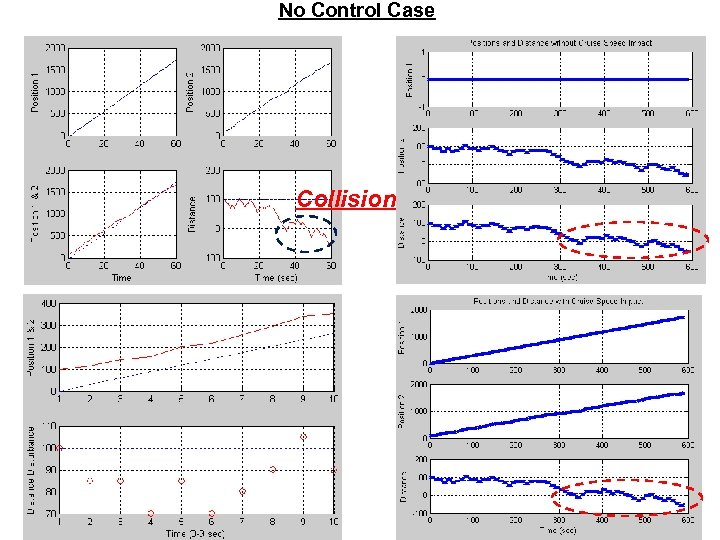No Control Case Collision!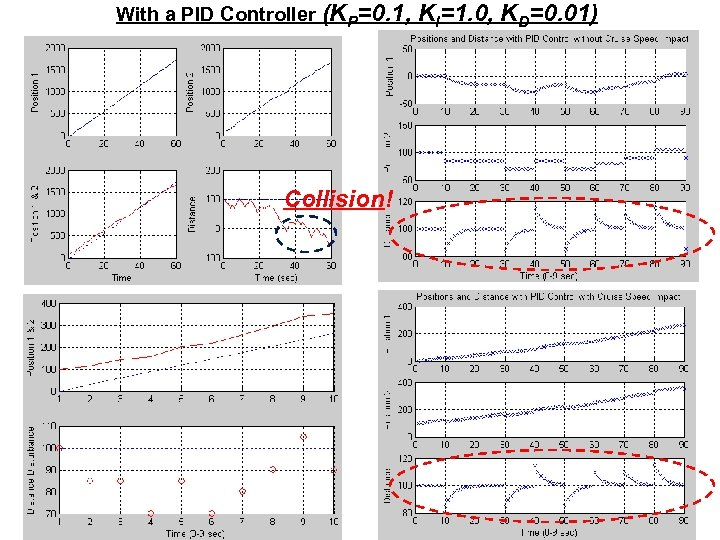With a PID Controller (KP=0. 1, Collision! KI=1. 0, KD=0. 01)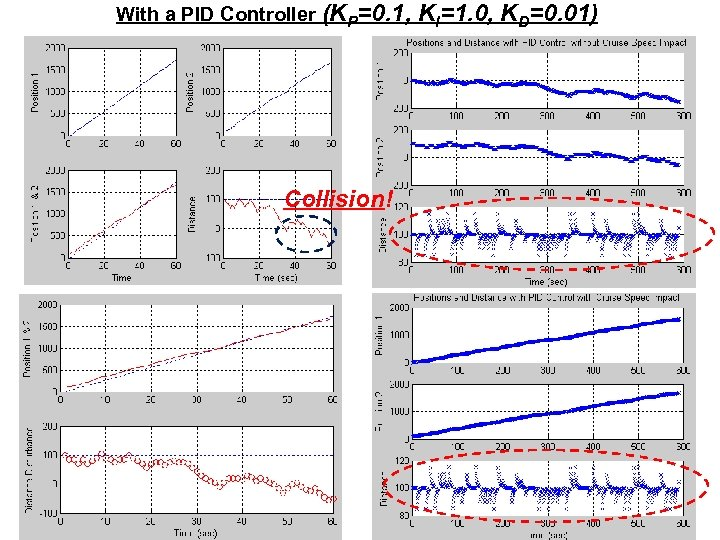With a PID Controller (KP=0. 1, Collision! KI=1. 0, KD=0. 01)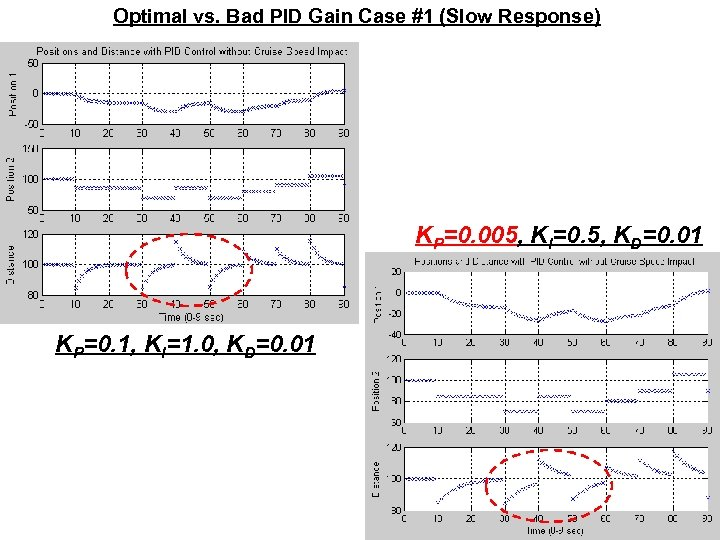Optimal vs. Bad PID Gain Case #1 (Slow Response) KP=0. 005, KI=0. 5, KD=0. 01 KP=0. 1, KI=1. 0, KD=0. 01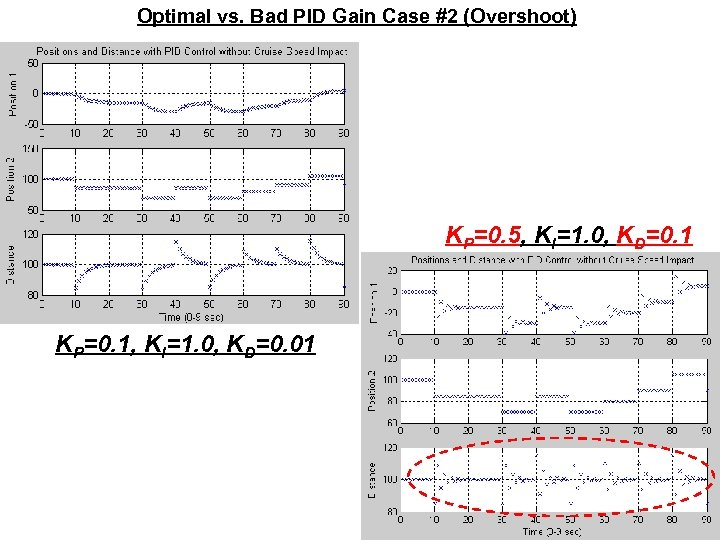Optimal vs. Bad PID Gain Case #2 (Overshoot) KP=0. 5, KI=1. 0, KD=0. 1 KP=0. 1, KI=1. 0, KD=0. 01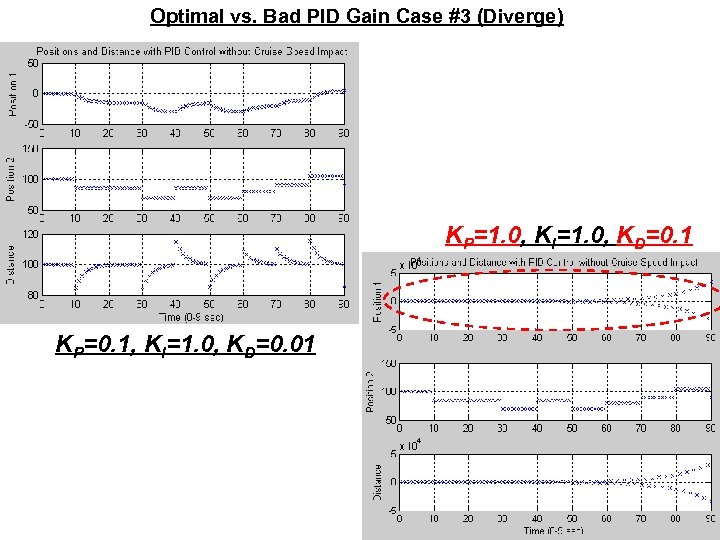Optimal vs. Bad PID Gain Case #3 (Diverge) KP=1. 0, KI=1. 0, KD=0. 1 KP=0. 1, KI=1. 0, KD=0. 01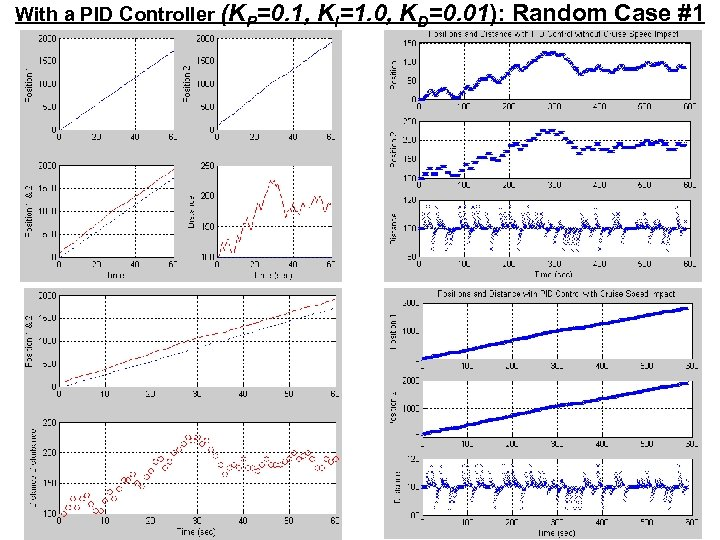With a PID Controller (KP=0. 1, KI=1. 0, KD=0. 01): Random Case #1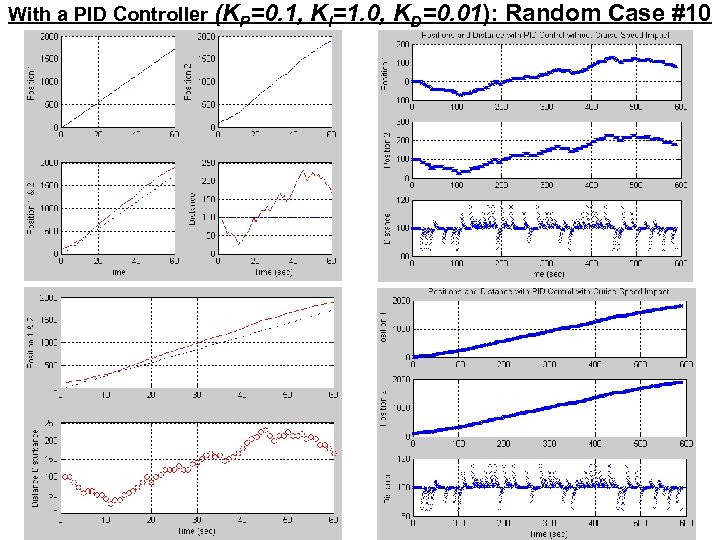With a PID Controller (KP=0. 1, KI=1. 0, KD=0. 01): Random Case #10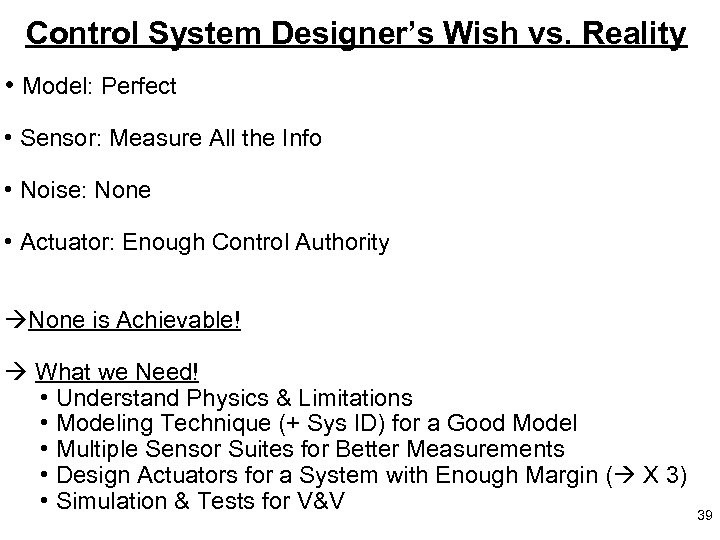Control System Designer’s Wish vs. Reality • Model: Perfect • Sensor: Measure All the Info • Noise: None • Actuator: Enough Control Authority None is Achievable! What we Need! • Understand Physics & Limitations • Modeling Technique (+ Sys ID) for a Good Model • Multiple Sensor Suites for Better Measurements • Design Actuators for a System with Enough Margin ( X 3) • Simulation & Tests for V&V 39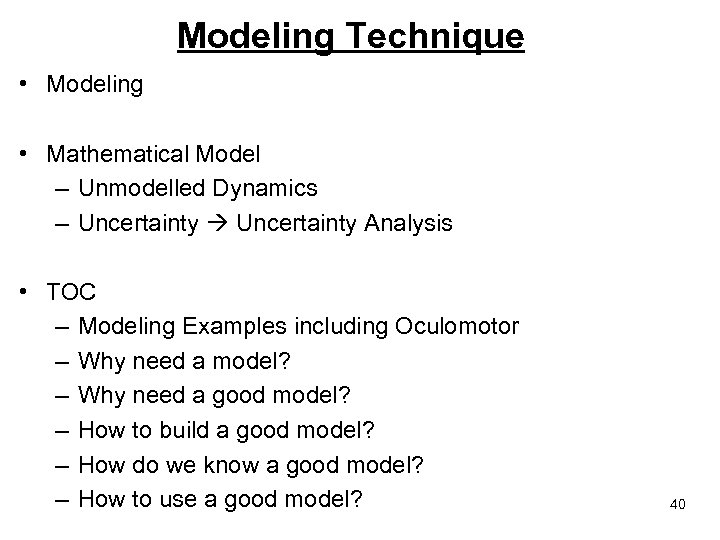Modeling Technique • Modeling • Mathematical Model – Unmodelled Dynamics – Uncertainty Analysis • TOC – Modeling Examples including Oculomotor – Why need a model? – Why need a good model? – How to build a good model? – How do we know a good model? – How to use a good model? 40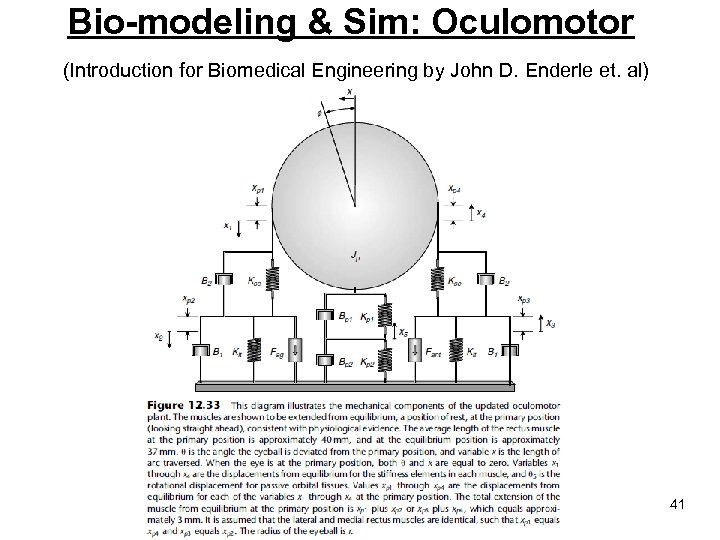Bio-modeling & Sim: Oculomotor (Introduction for Biomedical Engineering by John D. Enderle et. al) 41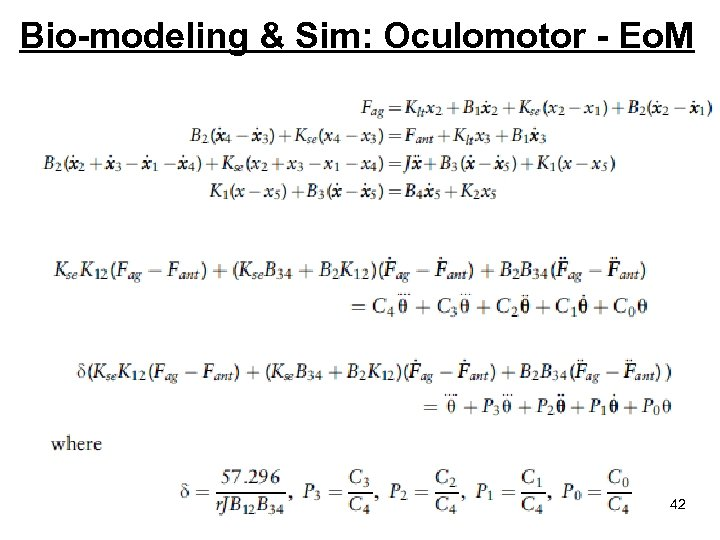Bio-modeling & Sim: Oculomotor - Eo. M 42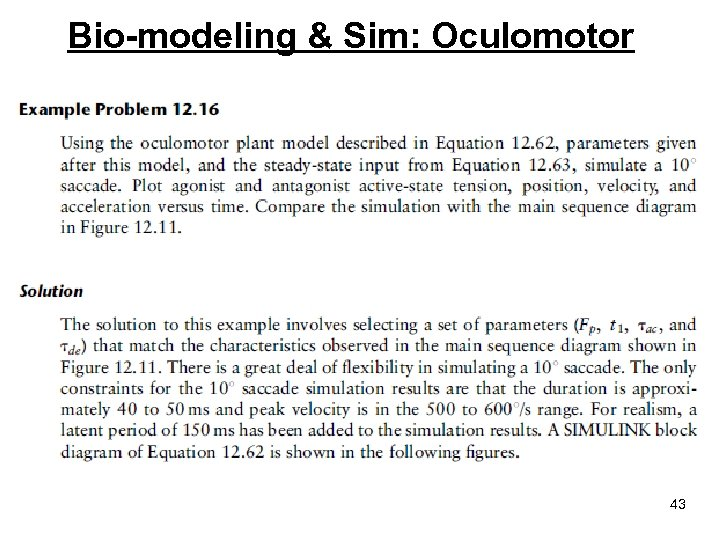Bio-modeling & Sim: Oculomotor 43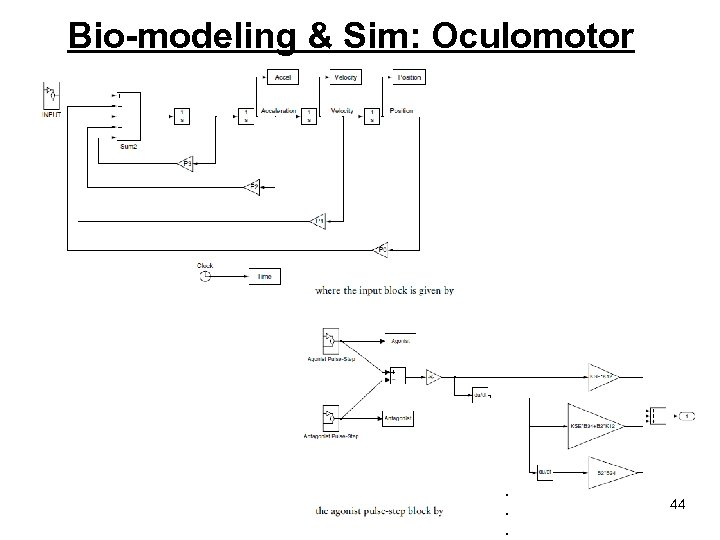Bio-modeling & Sim: Oculomotor . . . 44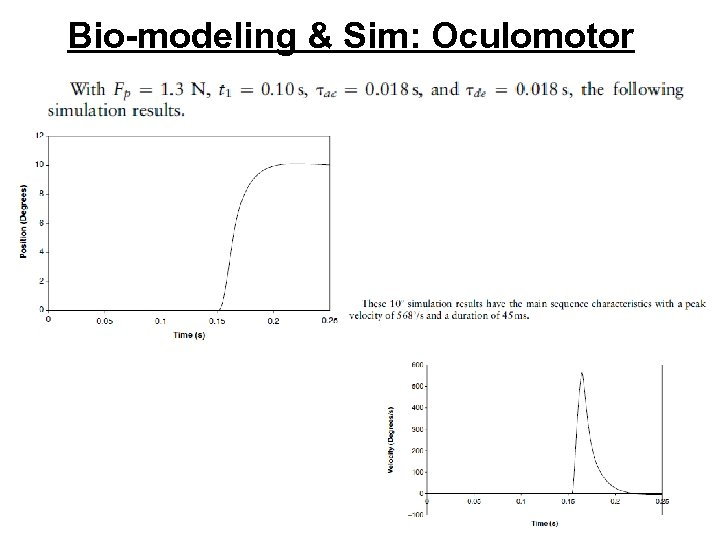Bio-modeling & Sim: Oculomotor 45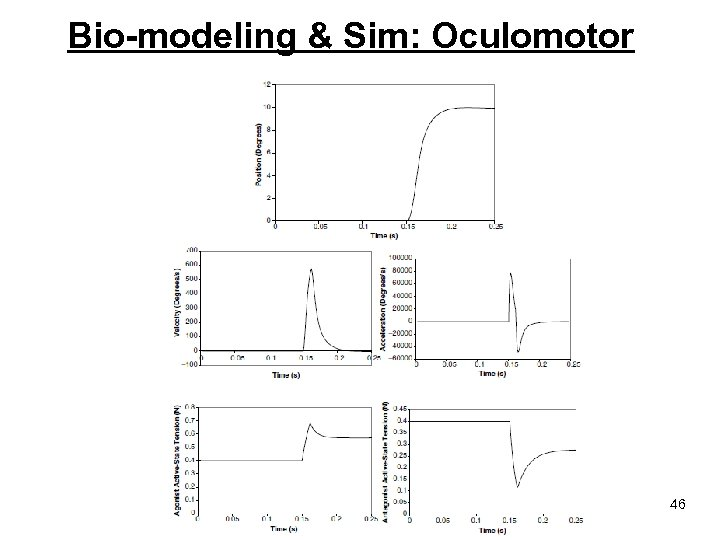Bio-modeling & Sim: Oculomotor 46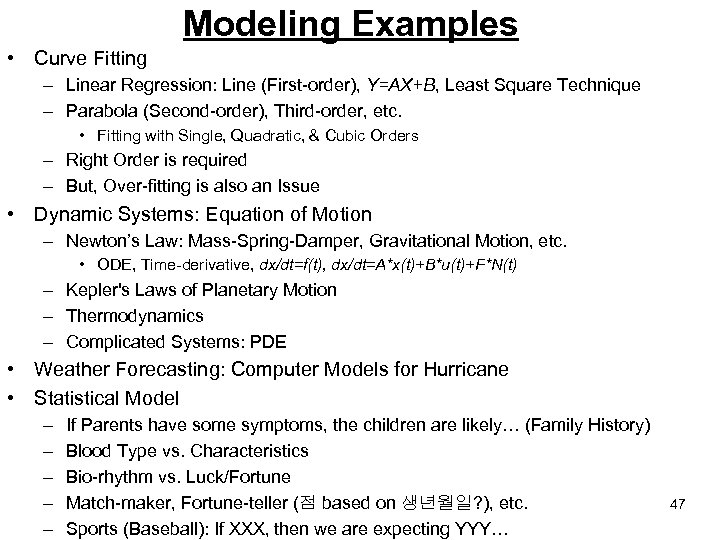Modeling Examples • Curve Fitting – Linear Regression: Line (First-order), Y=AX+B, Least Square Technique – Parabola (Second-order), Third-order, etc. • Fitting with Single, Quadratic, & Cubic Orders – Right Order is required – But, Over-fitting is also an Issue • Dynamic Systems: Equation of Motion – Newton’s Law: Mass-Spring-Damper, Gravitational Motion, etc. • ODE, Time-derivative, dx/dt=f(t), dx/dt=A*x(t)+B*u(t)+F*N(t) – Kepler's Laws of Planetary Motion – Thermodynamics – Complicated Systems: PDE • Weather Forecasting: Computer Models for Hurricane • Statistical Model – – – If Parents have some symptoms, the children are likely… (Family History) Blood Type vs. Characteristics Bio-rhythm vs. Luck/Fortune Match-maker, Fortune-teller (점 based on 생년월일? ), etc. Sports (Baseball): If XXX, then we are expecting YYY… 47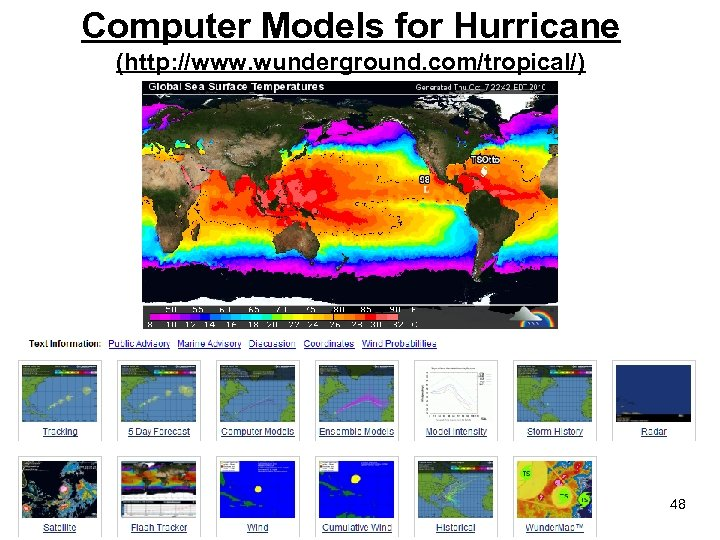Computer Models for Hurricane (http: //www. wunderground. com/tropical/) 48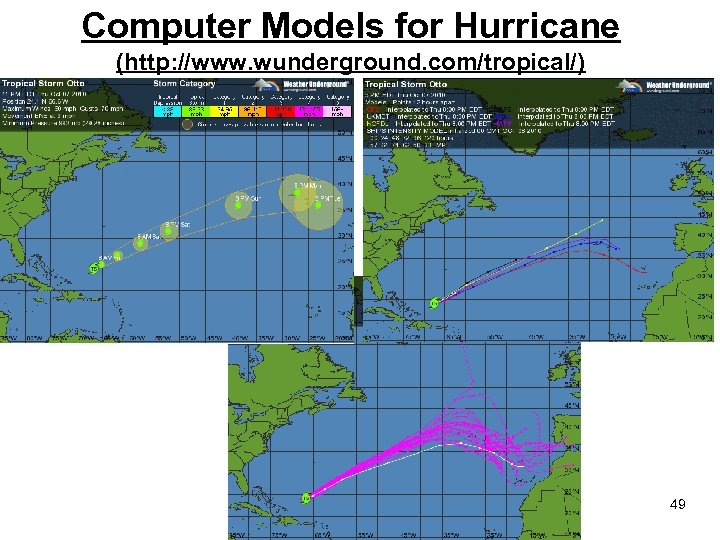Computer Models for Hurricane (http: //www. wunderground. com/tropical/) 49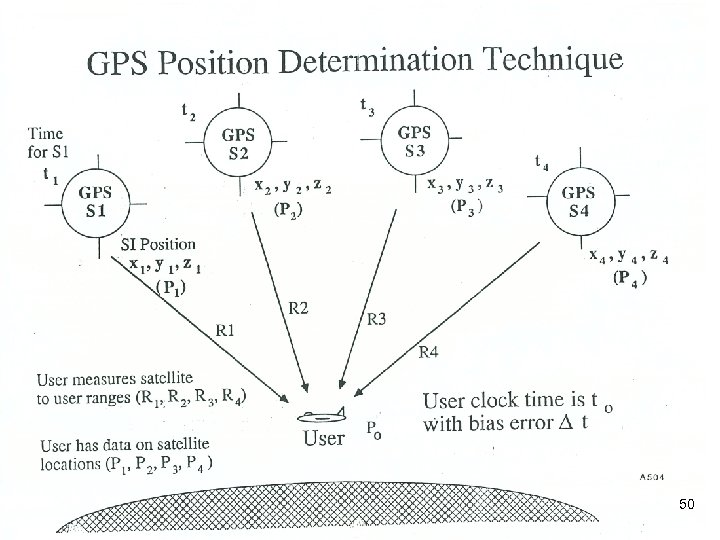50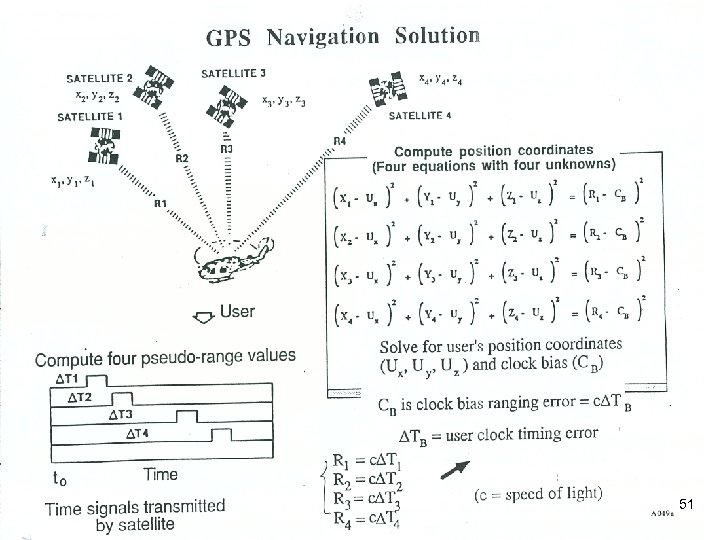51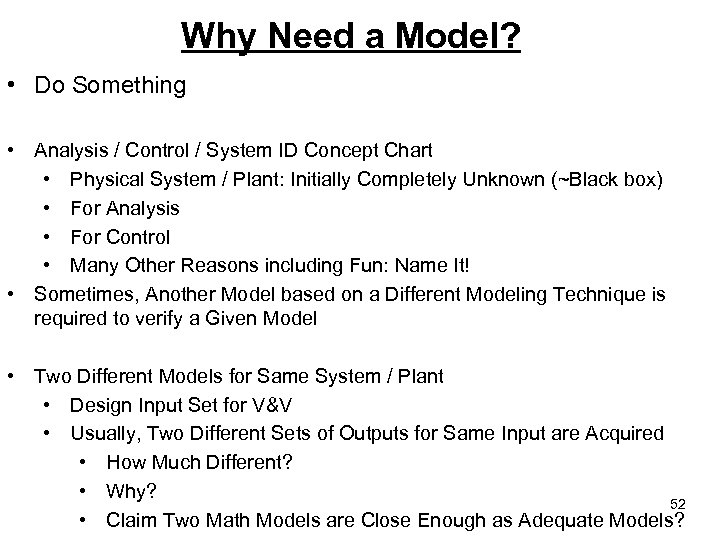Why Need a Model? • Do Something • Analysis / Control / System ID Concept Chart • Physical System / Plant: Initially Completely Unknown (~Black box) • For Analysis • For Control • Many Other Reasons including Fun: Name It! • Sometimes, Another Model based on a Different Modeling Technique is required to verify a Given Model • Two Different Models for Same System / Plant • Design Input Set for V&V • Usually, Two Different Sets of Outputs for Same Input are Acquired • How Much Different? • Why? 52 • Claim Two Math Models are Close Enough as Adequate Models?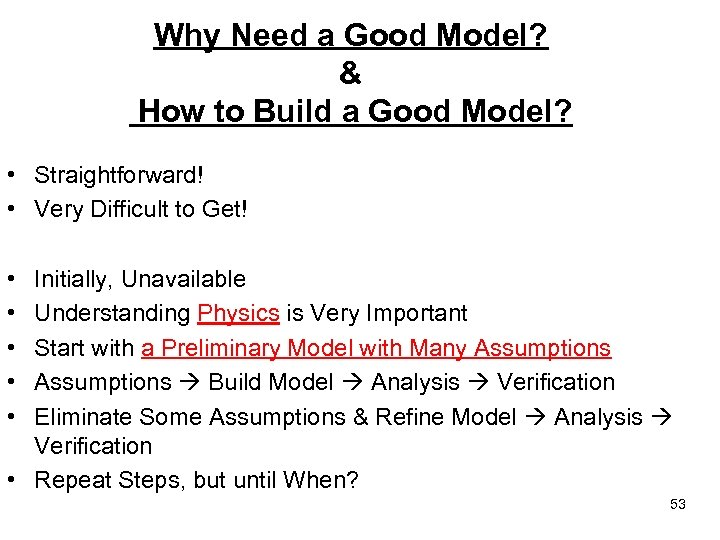Why Need a Good Model? & How to Build a Good Model? • Straightforward! • Very Difficult to Get! • • • Initially, Unavailable Understanding Physics is Very Important Start with a Preliminary Model with Many Assumptions Build Model Analysis Verification Eliminate Some Assumptions & Refine Model Analysis Verification • Repeat Steps, but until When? 53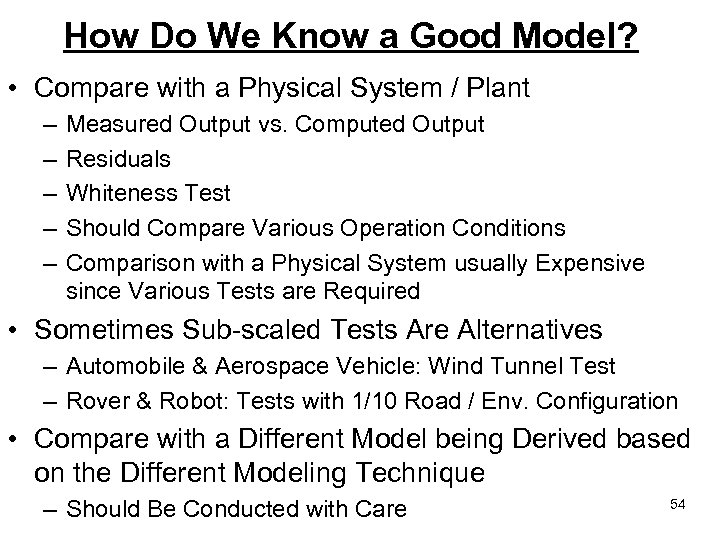How Do We Know a Good Model? • Compare with a Physical System / Plant – – – Measured Output vs. Computed Output Residuals Whiteness Test Should Compare Various Operation Conditions Comparison with a Physical System usually Expensive since Various Tests are Required • Sometimes Sub-scaled Tests Are Alternatives – Automobile & Aerospace Vehicle: Wind Tunnel Test – Rover & Robot: Tests with 1/10 Road / Env. Configuration • Compare with a Different Model being Derived based on the Different Modeling Technique – Should Be Conducted with Care 54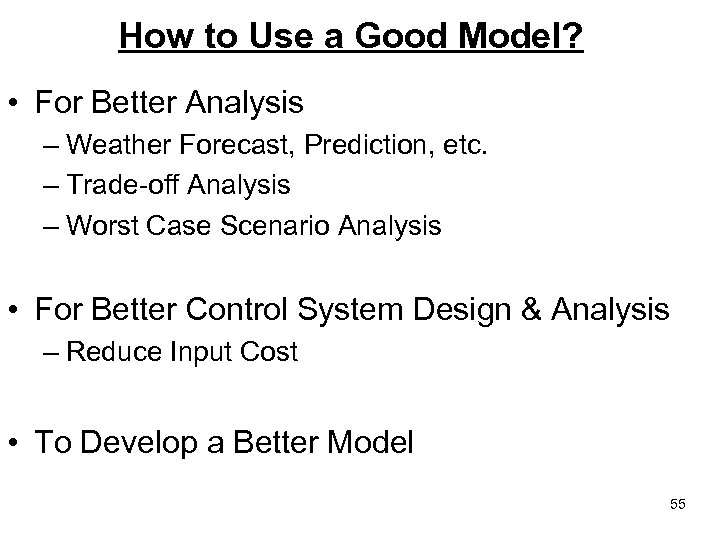How to Use a Good Model? • For Better Analysis – Weather Forecast, Prediction, etc. – Trade-off Analysis – Worst Case Scenario Analysis • For Better Control System Design & Analysis – Reduce Input Cost • To Develop a Better Model 55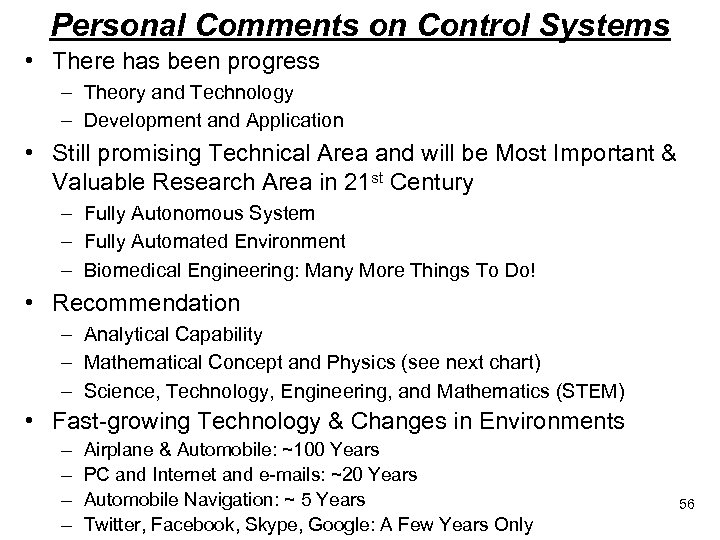Personal Comments on Control Systems • There has been progress – Theory and Technology – Development and Application • Still promising Technical Area and will be Most Important & Valuable Research Area in 21 st Century – Fully Autonomous System – Fully Automated Environment – Biomedical Engineering: Many More Things To Do! • Recommendation – Analytical Capability – Mathematical Concept and Physics (see next chart) – Science, Technology, Engineering, and Mathematics (STEM) • Fast-growing Technology & Changes in Environments – – Airplane & Automobile: ~100 Years PC and Internet and e-mails: ~20 Years Automobile Navigation: ~ 5 Years Twitter, Facebook, Skype, Google: A Few Years Only 56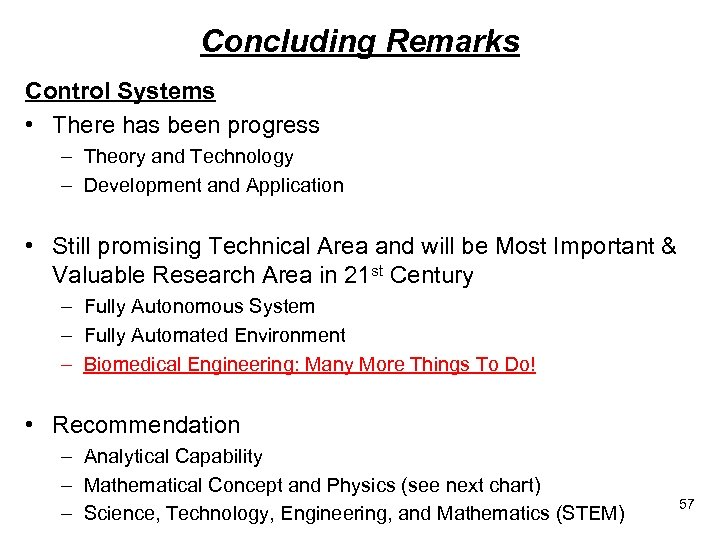Concluding Remarks Control Systems • There has been progress – Theory and Technology – Development and Application • Still promising Technical Area and will be Most Important & Valuable Research Area in 21 st Century – Fully Autonomous System – Fully Automated Environment – Biomedical Engineering: Many More Things To Do! • Recommendation – Analytical Capability – Mathematical Concept and Physics (see next chart) – Science, Technology, Engineering, and Mathematics (STEM) 57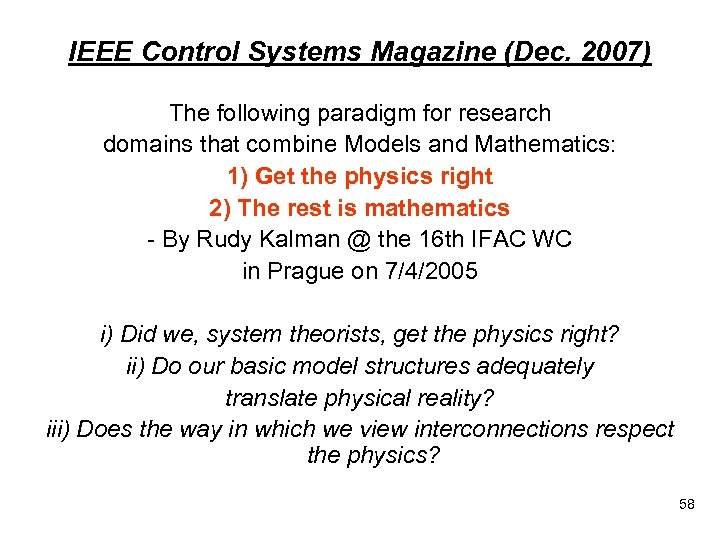IEEE Control Systems Magazine (Dec. 2007) The following paradigm for research domains that combine Models and Mathematics: 1) Get the physics right 2) The rest is mathematics - By Rudy Kalman @ the 16 th IFAC WC in Prague on 7/4/2005 i) Did we, system theorists, get the physics right? ii) Do our basic model structures adequately translate physical reality? iii) Does the way in which we view interconnections respect the physics? 58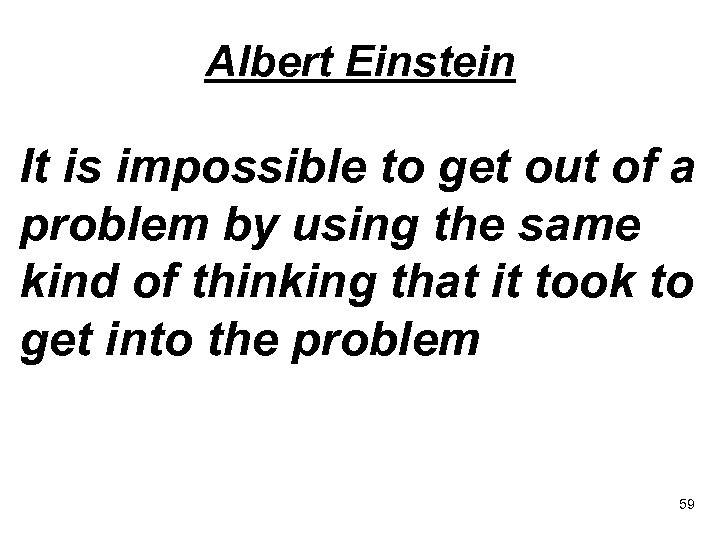Albert Einstein It is impossible to get out of a problem by using the same kind of thinking that it took to get into the problem 59THANK YOU! & Questions? [email protected] ac. kr 60